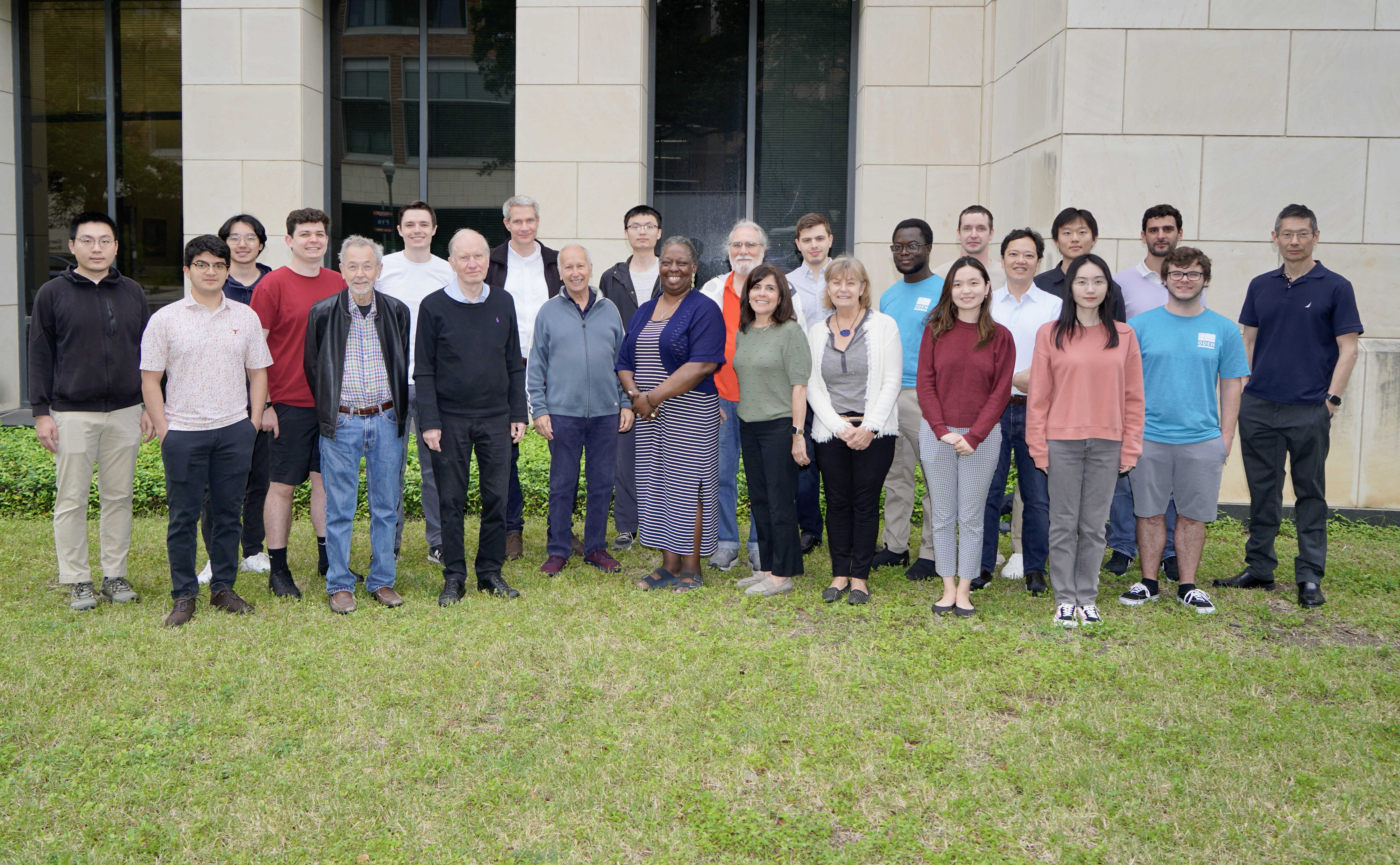# Applied Mathematics Group

Applied non-linear analysis, novel numerical methods and state of the arts applications. The Applied Mathematics Group (AMG) is an interdisciplinary research effort involving Mathematics, Statistics, Physics, and Computer Science. The group focuses on modeling of non-linear, coupled systems arising in the physical and biological sciences, engineering, medicine, and the social sciences. Model formulation, interpretation, approximation, and assessment are addressed, as well as issues such as mathematical well-posedness of models, multiple dimensional spatial and temporal scales in diverse geometrical configurations, direct and inverse problems, and accurate and efficient approximation algorithms. Classical and statistical analysis, data science including machine and deep learning methods, and numerical simulations by means of high-performance computing are used. This group interfaces with the activities of the Center of Numerical Analysis.

Website

Directors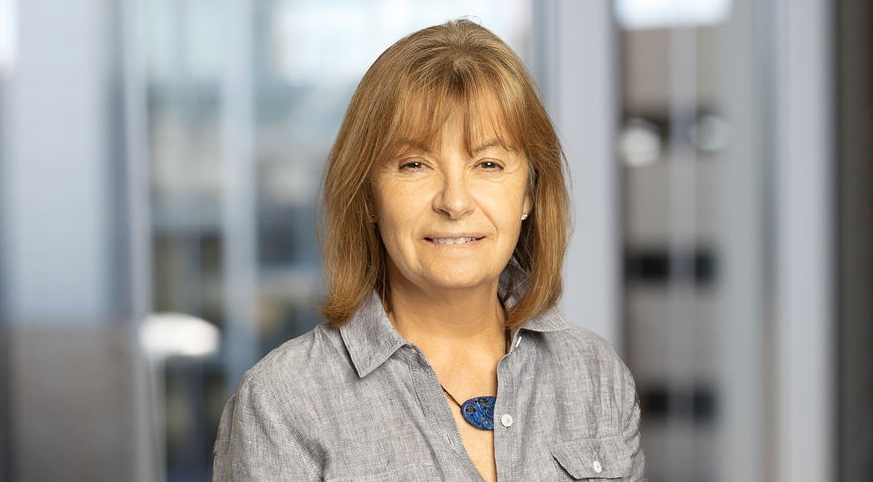###### Irene M. Gamba
Computational Statistical Mechanics Kinetic Theory Computational Physics

Faculty and Research Staff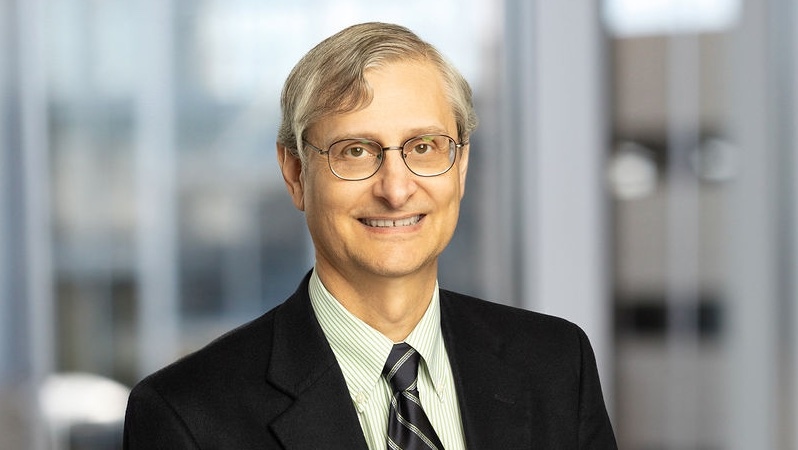###### Todd Arbogast
Numerical Analysis Computational Geosciences Applied Mathematics Partial Differential Equations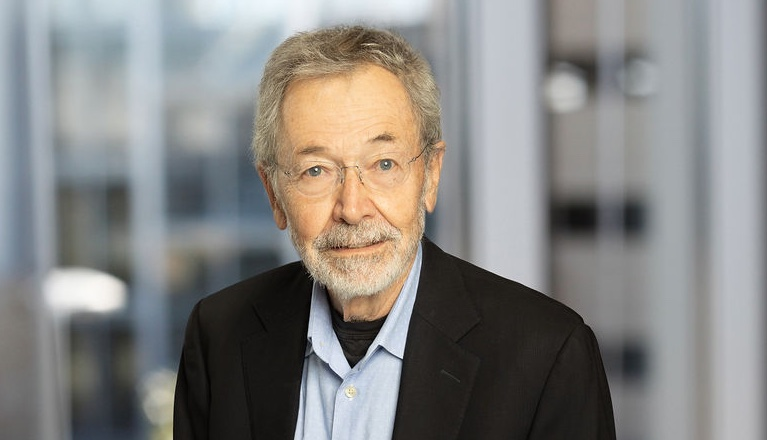###### William Beckner
Applied Mathematics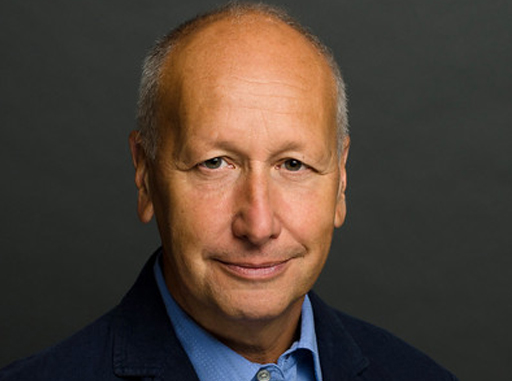###### Luis A. Caffarelli
Applied Mathematics Partial Differential Equations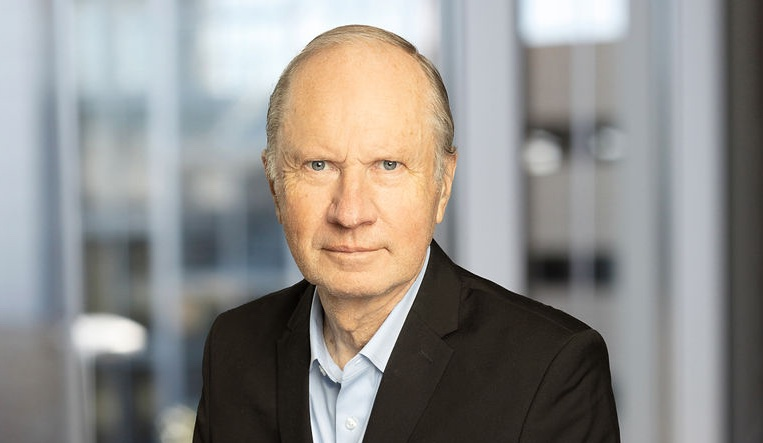###### Bjorn Engquist
Numerical Analysis Applied Mathematics###### Oscar Gonzalez
Computational Mechanics Applied Mathematics Differential Equations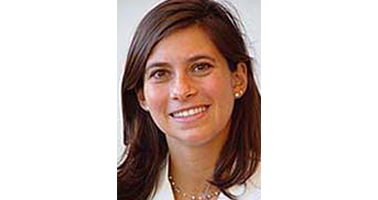###### Lauren Meyers
Computational Biology Data Science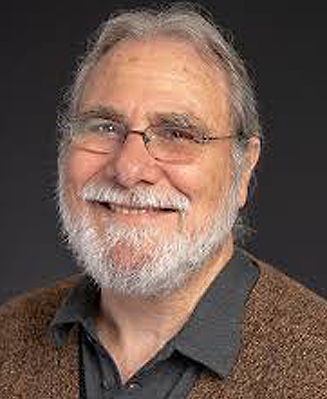###### Philip J. Morrison
Computational Statistical Mechanics Computational Physics Computational Mechanics High-Performance Computing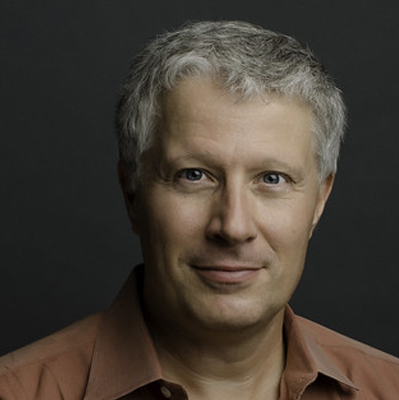###### Peter Mueller
Uncertainty Quantification Computational Statistical Mechanics Bayesian Methods Simulation techniques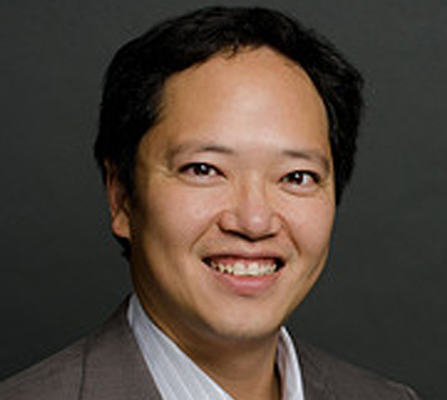###### Richard Tsai
Machine Learning Numerical Analysis Scientific Computing Partial Differential Equations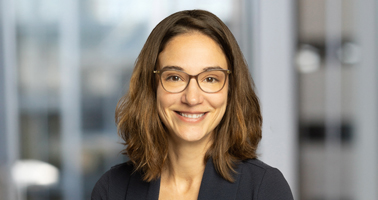###### Rachel Ward
Uncertainty Quantification Optimization Data Science

Students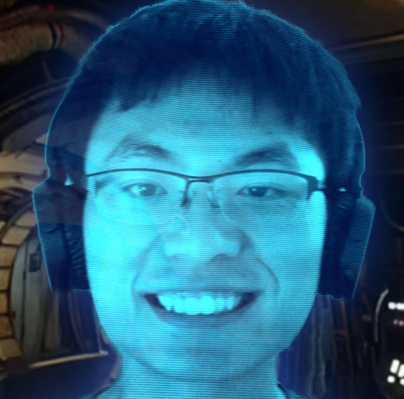###### Kun Huang

Staff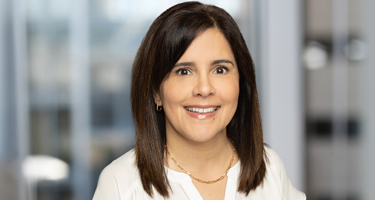###### Melibea Jacaman

Members outside the Oden Institute

William Porteous